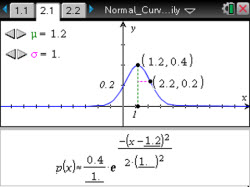# Activities

•• ##### Subject Area

• Math: Statistics: Normal Distributions

• ##### Author9-12

45 Minutes

• ##### Device
•TI-Nspire™ CX/CX II
•TI-Nspire™ CX CAS/CX II CAS
• TI-Nspire™ Navigator™
• TI-Nspire™
• TI-Nspire™ CAS
• ##### Software

TI-Nspire™
TI-Nspire™ CAS

3.0

## Exploring the Normal Curve Family#### Activity Overview

Students will investigate the relationship of the equation of a normal curve to its graph. They will use a slider to change the values of two parameters, m and s, to investigate their effects on the normal curve, noting in particular that m represents the location of the mean and that s represents the distance from the mean to the curve at the point of inflection.

#### Objectives

• Students will identify the three defining characteristics of a normal curve related to shape, center, spread, and area.
• Students will recognize that normal curves form a family whose members share these same characteristics.
• Students will use appropriate tools strategically (CCSS Mathematical Practices).
• Students will model with mathematics (CCSS Mathematical Practices).

#### Vocabulary

• normal curve
• mean
• standard deviation
• point of inflection
• density functions